# Chapter 6.1. Nested Loops

In the current chapter, we will be looking at nested loops and how to use for loops to draw various figures on the console, that contain symbols and signs, ordered in rows and columns on the console. We will use single, and nested loops (loops that stay in other loops), calculations and checks, to print on the console simple and not so simple figures by specified sizes.

### Problem: Rectangle of 10 x 10 Stars

Print on the console a rectangle made out of 10 x 10 stars.

Input Output
(None) **********
**********
**********
**********
**********
**********
**********
**********
**********
**********

#### Hints and Guidelines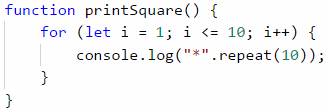How does the example work? We initialize a loop with a variable i = 1, which increases with each iteration of the loop, while it is less or equal to 10. This way the code in the body of the loop is executed 10 times. In the body of the loop, we print a new line on the console "*".repeat(10), which creates a string of 10 stars.

### Example: Rectangle Made of N x N Stars

Write a program that gets a positive integer n and prints on the console a rectangle made out of N x N stars.

Input Output Input Output Input Output
2 **
**
3 ***
***
***
4 ****
****
****
****

#### Hints and Guidelines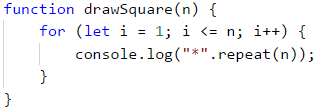#### Note

On some web browsers, the repeating results on the console merge in one. It's advised to use NodeJS for our current examples. If you still get to this case you can use the symbol \n at the end of the printing method console.log("*".repeat(10) + "\n");.

## Nested Loops

A nested loop is a construction where the body of one loop (the outer one) stays inside another loop (the inner one). In each iteration of the outer loop, the whole inner loop is executed. This happens in the following way:

• When nested loops start executing, the outer loop starts first: the controlling variable is initialized and after a check for ending the loop the code in its body is executed.
• After that, the inner loop is executed. The controlling variables' start position is initialized, a check for ending the loop is made and the code in its body is executed.
• When reaching the specified value for ending the loop, the program goes back one step up and continues executing the previous outer loop. The controlling variable of the outer loop changes with one step, a check is made to see if the condition for ending the loop is met and a new execution of the nested (inner) loop is started.
• This is repeated until the variable of the outer loop meets the condition to end the loop.

Here is an example that illustrates nested loops. The aim is again to print a rectangle made of n * n stars, in which for each row a loop iterates from 1 to n, and for each column a nested loop is executed from 1 to *n:

function drawSquare(n) {
for (let i = 1; i <= n; i++) {
let stars = "";

for (let j = 1; j <= n; j++) {
stars += "*";
}

console.log(stars);
}
}


Let's look at the example above. After initializing the first (outer) loop, its body, which contains the second (nested) loop starts executing. By itself, it prints on one row n number of stars. After the inner loop finishes executing at the first iteration of the outer one, the first loop will continue, i.e. it will print an empty row on the console. After that, the variable of the first loop will be renewed and the whole second loop will be executed again. The inner loop will execute as many times as the body of the outer loop executes, in this case, n times.

### Problem: Rectangle of N x N Stars

Print on the console a square made of N x N stars (use a space between the stars, staying on the same line):

Input Output Input Output Input Output
2 * *
* *
3 * * *
* * *
* * *
4 * * * *
* * * *
* * * *
* * * *

#### Hints and Guidelines

The problem is similar to the last one. The difference here is that we need to figure out how to add a whitespace after the stars so that there aren't any excess white spaces at the beginning and the end.### Problem: Square of Stars

Write a program that draws on the console a square of N x N asterisks:

Input Output Input Output Input Output
2 * *
* *
3 * * *
* * *
* * *
4 * * * *
* * * *
* * * *
* * * *

#### Hints and Guidelines

The problem is similar to the previous one. Here, it is necessary to consider how to print a space after the asterisks so that there are no unnecessary spaces at the beginning of the end.### Problem: Triangle of Dollars

Write a program that takes an integer n and prints a triangle made of dollars of size n.

Input Output Input Output Input Output
3 $ $ $ $ 
4 $ $ $ $ 
 
5 $ $ $ $ 
 
  \$

#### Hints and Guidelines

The problem is similar to those for drawing a rectangle and square. Once again, we will use nested loops, but there is a catch here. The difference is that the number of columns that we need to print depends on the row, on which we are and not on the input number n. From the example input and output data, we see that the count of dollars depends on which row we are on at the moment of the printing, i.e. 1 dollar means the first row, 3 dollars mean the third row, and so on. Let's see the following example in detail. We see that the variable of the nested loop is connected with the variable of the outer one. This way our program prints the desired triangle.### Problem: Square Frame

Write a program that takes a positive integer n and draws on the console a square frame with a size of n * n.

Input Output Input Output
3 + - +
| - |
+ - +
4 + - - +
| - - |
| - - |
+ - - +
Input Output Input Output
5 + - - - +
| - - - |
| - - - |
| - - - |
+ - - - +
6 + - - - - +
| - - - - |
| - - - - |
| - - - - |
| - - - - |
+ - - - - +

#### Hints and Guidelines

We can solve the problem in the following way:

• We read from the console the number n.
• We print the upper part: first a + sign, then n-2 times - and in the end a + sign.
• We print the middle part: we print n-2 rows, as we first print a | sign, then n-2 times - and in the end again a | sign. We can do this with nested loops.
• We print the lower part: first a + sign, then n-2 times - and in the end a + sign.

Here is an example implementation of the above idea with nested loops: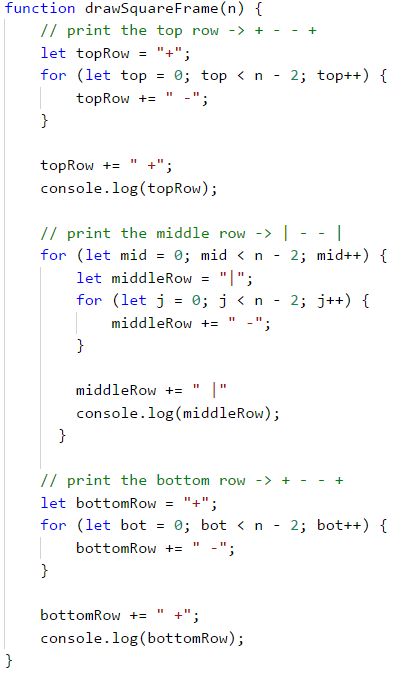### Problem: Rhombus of Stars

Write a program that takes a positive integer n and prints a rhombus made of stars with size n.

Input Output Input Output
1 * 2  *
* *
 *
Input Output Input Output
3   *
 * *
* * *
 * *
  *
4    *
  * *
 * * *
* * * *
 * * *
  * *
   *

#### Hints and Guidelines

To solve this problem, we need to mentally divide the rhombus into two parts – the upper one, which also includes the middle row, and the lower one. For the printing of each part, we will use two separate loops, as we leave the reader to decide the dependency between n and the variables of the loops. For the first loop we can use the following guidelines:

• We print n-row white spaces.
• We print *.
• We print row-1 times *.

The second (lower) part will be printed similarly, which again we leave to the reader to do.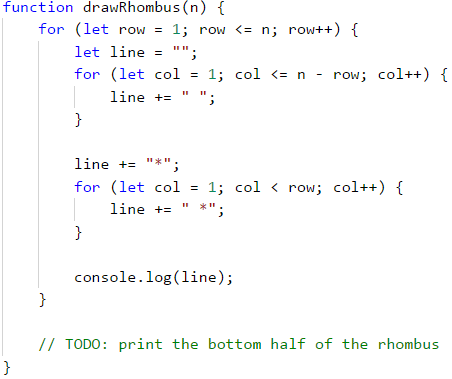### Problem: Christmas Tree

Write a program that takes a number n (1 ≤ n ≤ 100) and prints a Christmas tree with a height of n+1.

Input Output Input Output
1   |
* | *
2    |
 * | *
** | **
Input Output Input Output
3     |
  * | *
 ** | **
*** | ***
4      |
   * | *
  ** | **
 *** | ***
**** | ****

#### Hints and Guidelines

From the examples, we see that the Christmas tree can be divided into three logical parts. The first part is the stars and the white spaces before and after them, the middle part is |, and the last part is again stars, but this time there are white spaces only before them. The printing can be done with only one loop and the .repeat(n) method, which we will use once for the stars and once for the white spaces: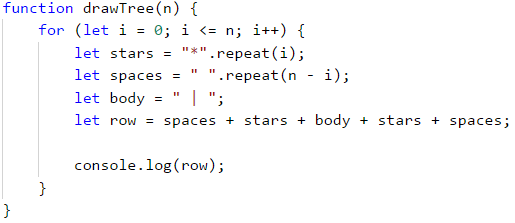## Exercises: Drawing more complex figures

Let's look at how to draw figures using nested loops with more complex logic, for which we need to think more before coding.

### Problem: Sunglasses

Write a program that takes an integer n (3 ≤ n ≤ 100) and prints sunglasses with a size of 5*n x n as found in the examples:

Input Output Input Output
3 ******   ******
*////*|||*////*
******   ******
4 ********    ********
*//////*||||*//////*
*//////*    *//////*
********    ********
Input Output
5 **********     **********
*////////*     *////////*
*////////*|||||*////////*
*////////*     *////////*
**********     **********

#### Hints and Guidelines

From the examples, we can see that the sunglasses can be divided into three parts – upper, middle, and lower. A part of the code with which the problem can be solved is given below. When drawing the upper and lower rows we need to print 2 * n stars, n white spaces, and 2 * n stars: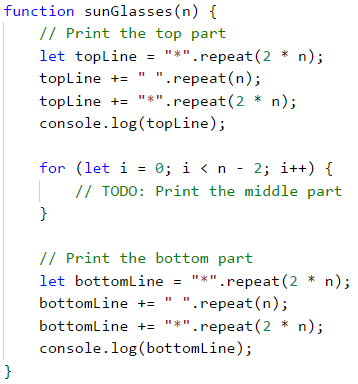When drawing the middle part, we need to check if the row is (n-1) / 2 - 1, because in the examples we can see that in this row we need to print pipes instead of white spaces. The problem with (n - 1) / 2 - 1 is that it can be a number with the decimal remainder. Because of this, we must use a mathematical method for removing a decimal remainder - Math.floor(...). Math.floor(...) returns the bigger number, which is smaller or equal to the last number: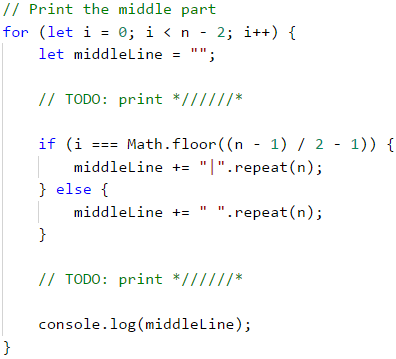### Problem: House

Write a program that takes a number n (2 ≤ n ≤ 100) and prints a house with size n x n, just as in the examples:

Input Output Input Output Input Output
2 **
||
3 -*-
***
|*|
4 -**-
****
|**|
|**|
Input Output Input Output
5 --*--
-***-
*****
|***|
|***|
8 ---**---
--****--
-******-
********
|******|
|******|
|******|
|******|

#### Hints and Guidelines

We understand from the problem explanation that the house is with a size of n x n. What we see from the example input and output is that:

• The house is divided into two parts: roof and base.• When n is an even number, the point of the house is "dull".
• When n is odd, the roof is one row larger than the base.
##### The Roof
• It comprises stars and dashes.
• In the top part, there are one or two stars, depending on if n is even or odd (also related to the dashes).
• In the lowest part, there are many stars and no dashes.
• With each lower row, the stars increase by 2, and the dashes decrease by 2.
##### The Base
• The height is n rows.
• It is made out of stars and pipes.
• Each row comprises 2 pipes – one in the beginning and one at the end of the row, and also stars between the pipes with a string length of n - 2.

We gave n as a parameter to our function:It is very important to check if the input data is correct! In these tasks, it is not a problem to directly convert the data from the console into Numbertype, because it is said that we will be given valid integers. If you are making more complex programs it is a good practice to check the data. What will happen if instead of the character "A" the user inputs a number?

To draw the roof, we write down how many stars we start with a variable called stars:

• If n is an even number, there will be 2 stars.
• If it is odd, there will be 1.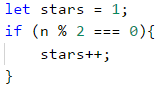Calculate the length of the roof. It equals half of n. Write the result in the variable roofLength.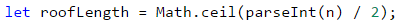It is important to note that when n is an odd number, the length of the roof is one row more than that of the base. In JavaScript language, when you divide two numbers with a remainder, the result will be a decimal number Example:

    let result = 3 / 2; // result 1.5


If we want to round up to the next largest integer. number, we need to use the method Math.ceil(…): let result = Math.ceil(3 / 2);. The result from 3 / 2 is 1.5. Math.ceil(…) will round the number to the next largest integer. In our case 1.5 will be rounded to 2. parseInt() is used to transform the input parameter to type Number.

After we have calculated the length of the roof, we make a loop from 0 to roofLength. On each iteration we will:

• Calculate the number of dashes we need to draw. The number will be equal to (n - stars) / 2. We store it in variable padding.• We print on the console: "dash" (padding / 2 times) + "stars" (stars times) + "dash" (padding / 2 times):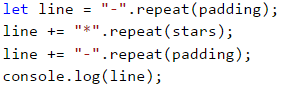• Before the iteration is over, we add 2 to stars (the number of the stars).After we have finished with the roof, it is time for the base. It is easier to print:

• We start with a loop from 0 to n (not inclusive).
• We print on the console: | + * (n - 2 times) + |.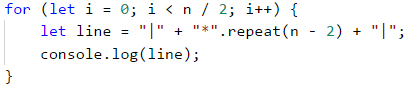If you have written everything as it is here, the problem should be solved.

### Problem: Diamond

Write a program that takes an integer n (1 ≤ n ≤ 100) and prints a diamond with size n, as in the following examples:

Input Output Input Output Input Output
1 *
2 ** 3 -*-
*-*
-*-
Input Output Input Output Input Output
4 -**-
*--*
-**-
5 --*--
-*-*-
*---*
-*-*-
--*--
6 --**--
-*--*-
*----*
-*--*-
--**--
Input Output Input Output Input Output
7 ---*---
--*-*--
-*---*-
*-----*
-*---*-
--*-*--
---*---
8 ---**---
--*--*--
-*----*-
*------*
-*----*-
--*--*--
---**---
9 ----*----
---*-*---
--*---*--
-*-----*-
*-------*
-*-----*-
--*---*--
---*-*---
----*----

#### Hints and Guidelines

What we know from the problem explanation is that the diamond is with size n x n.

From the example input and output we can conclude that all rows contain exactly n symbols, and all the rows, except for the top and bottom ones, have 2 stars. We can mentally divide the diamond into 2 parts:

• Upper part. It starts from the upper tip down to the middle.
• Lower part. It starts from the row below the middle one and goes down to the lower tip (inclusive).
##### Upper Part
• If n is an odd number, it starts with 1 star.
• If n is an even number, it starts with 2 stars.
• With each row down, the stars get further away from each other.
• The space between, before, and after the stars is filled up with dashes.
##### Lower Part
• With each row down, the stars get closer to each other. This means that space (the dashes) between them is getting smaller and space (the dashes) on the left and the right is getting larger.
• The bottom-most part has 1 or 2 stars, depending on whether n is an even or odd number.
##### Upper and Lower Parts of the Diamond
• On each row, except the middle one, the stars are surrounded by inner and outer dashes.
• On each row, there is space between the two stars, except on the first and the last row (sometimes the star is 1).

As an entering parameter n of our function we give it a Number value:We start drawing the upper part of the diamond. The first thing we need to do is to calculate the number of the outer dashes leftRight (the dashes on the outer side of the stars). It is equal to (n - 1) / 2, rounded down. To round the number we will use the method Math.floor(...) to remove the residue. We can have this case if our input is odd.After we have calculated leftRight, we start drawing the upper part of the diamond. We can start by running a loop from 0 to n / 2 + 1 (rounded down). At each iteration of the loop the following steps must be taken:

• We draw on the console the left dashes (with length leftRight) and right after them the first star:• We will calculate the distance between the two stars. We can do this by subtracting from n the number of the outer dashes, and the number 2 (the number of the stars, i.e. the diamond's outline). We need to store the result of the subtraction in a variable mid.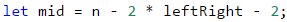• If the mid is lower than 0, we know that on the row there should be only 1 star. If it is higher or equal to 0 then we have to print dashes with length mid and one star after them.

• We draw on the console the right outer dashes with length leftRight:• At the end of the loop, we decrease leftRight by 1 (the stars are moving away from each other).

We are ready with the upper part.

Printing the lower part is very similar to that of the upper part. The difference is that instead of decreasing leftRight with 1 at the end of the loop, we will increase leftRight with 1 at the beginning of the loop. Also, the loop will be from 0 to (n - 1) / 2.Repeating a code is considered bad practice because the code becomes very hard to maintain. Let's imagine that we have a piece of code (e.g. the logic for drawing a row from the diamond) at a few more places and we decide to change it. For this, we will have to go through all the places and change it everywhere. Now let's imagine that you need to reuse a piece of code not 1, 2, or 3 times but tens of times. A way to overcome this problem is to use functions. You can look for additional information for functions on the Internet or look at Chapter “10” (Functions).

If we have written all correctly, then the problem is solved.

## What Have We Learned from This Chapter?

We learned how to use the repeat(...) method which constructs and returns a new object from type String:

let foo = "*".repeat(10);


We learned how to draw figures using nested for loops:

for (let i = 1; i <= n; i++) {
let stars = "";

for (let j = 1; j <= n; j++) {
stars += "*";
}

console.log(stars);
}


## Lab: Drawing Ratings in Web

Now that we got used to nested loops and the way to use them to draw figures on the console, we can get into something even more interesting: we can see how loops can be used to draw in a Web environment. We will make a web application that visualizes a number rating (a number from 0 to 100) with stars. This kind of visualization is common in e-commerce sites, reviews of products, event rating, rating of apps, and others.

Don't worry if you don't understand all of the code, how exactly it is written and how the project works. It is normal, now we are learning to write code and we are a long way from the web development technologies. If you are struggling to write your project by following the steps, watch the video from the beginning of the chapter or ask for help in the SoftUni official discussion Reddit: https://www.reddit.com/r/softuni/.

### Problem: * Ratings – Visualization in a Web Environment

Your task is to create a JavaScript web application for visualizing a rating (a number from 0 to 100). From 1 to 10 stars should be drawn with halves. The starts should be generated with a for loop.

We create an empty folder in our file system with the name "ratings". In there we create 2 files and one folder:

• index.html
• script.js
• images (folder)

Now we add images with stars (they are part of the resources of this lab which can be downloaded from here). We copy them from windows explorer and paste them into the folder images using copy/paste.

We open index.html and add the following code:This code creates one input field input-rating, in which the user can add a number from [0 … 100] and a button [Draw] which when clicked calculates the value of the stars with their input value. The action which will approve the input data is called drawRating. After that the form will print the content of <div id="ratingHolder"></div>. The code which is inside it will be dynamically generated HTML with a series of stars.

We add the function drawRating() inside the file script.js, which has the following code:

/**
* drawRating, draws HTML, which is needed for the visualization of the stars
* @param {Number} rating
* @return {String} html
*/
function drawRating(rating) {
// string from HTML
let html = "";

// total number of stars
let allStars = 10;

// all field stars
let fullStars = Math.floor(rating / allStars);

// all empty stars
let emptyStars = Math.floor((100 - rating) / allStars);

// all half-filled stars
let halfStars = allStars - fullStars - emptyStars;

// build of HTML
for (let i = 0; i < fullStars; i++) {
html += '<img src="images/full-star.png">';
}
for (let i = 0; i < halfStars; i++) {
html += '<img src="images/half-star.png">';
}
for (let i = 0; i < emptyStars; i++) {
html += '<img src="images/empty-star.png">';
}

// return of created HTML
return html;
}


The code above takes the number rating, makes some calculations to find the number of empty stars and the number of half-full stars, after which it generates an HTML code, which orders a few pictures of stars one after the other so that it can make the rating picture from them. The ready HTML code is returned as a result of the function and it is ready for further use. Up to this moment, the result from this point can't be used because we can't connect it to the button. We create a function named drawHandler() with the following code:

/**
* drawHandler, a function that runs, when the user clicks on the button Draw
* @return {Void}
*/
function drawHandler() {
// Finds the input element, which stores the raiting and get its value
let ratingInput = document.getElementById("input-rating");

// By default all values from the form come as "string"
// This is why we need to convert them using "parseInt()"
let rating = parseInt(ratingInput.value);

// We find the element which holds the stars
let ratingHolder = document.getElementById("ratingHolder");

// Generated HTML from our input rating
let html = drawRating(rating);

// draw of a page
ratingHolder.innerHTML = html;
}


Our function drawHandler() makes several things:

• Finds the HTML element, which holds the rating (input-rating) and gets its value.
• Converts value from string to a number.
• Finds the HTML element that will hold the stars (ratingHolder).
• Generates HTML of the stars, using drawRating(...) function.
• Places the generated HTML using innerHTML method inside the element ratingHolder.

We need one more function that will combine the above 2 functions and connect them to the HTML elements. This function is called appInit(). The name hints that its role will be to initialize our application. We add the following code in our function appInit():

/**
* appInit is responsible for our initial run of the application
* @return {Void}
*/
function appInit() {
// finds the button element inside our HTML
let button = document.getElementById("input-draw");

// Adds "click" event to perform the drawing

// Initial draw of the rating
drawHandler();
}


After we have all the needed functions it's time to start our application. Keep in mind that script.js is added at the end of our file (after the closing tag of </body>). This is a good practice and gives us faster loading of the DOM tree. This allows us to run the following JavaScript code, which uses HTML elements. Because of these conditions, we can be sure that all conditions are already loaded inside the memory of our browser.

However, instead of calling directly appInit() at the end of the file, we will use one more good practice:

/**
* Stars the application asynchronous using "event listener".
*/


The event DOMContentLoaded confirms that the browser has finished will all actions connected to the creation of the DOM tree. Adding to it using addEventListener(...) provides it with a correct run of our JavaScript program.

When the browser is ready, it will run our starting function appInit(). The result from our function is:

• Hooks our function drawHandler() to the click event of the Draw button.
• Initial call of drawHandler() is to fill the stars from our current HTML.

If you have a problem with the project above watch the video at the beginning of this chapter. Inside the video, the application is made live step by step with a lot of explanations. You can also ask in the Softuni Reddit: https://www.reddit.com/r/softuni/.